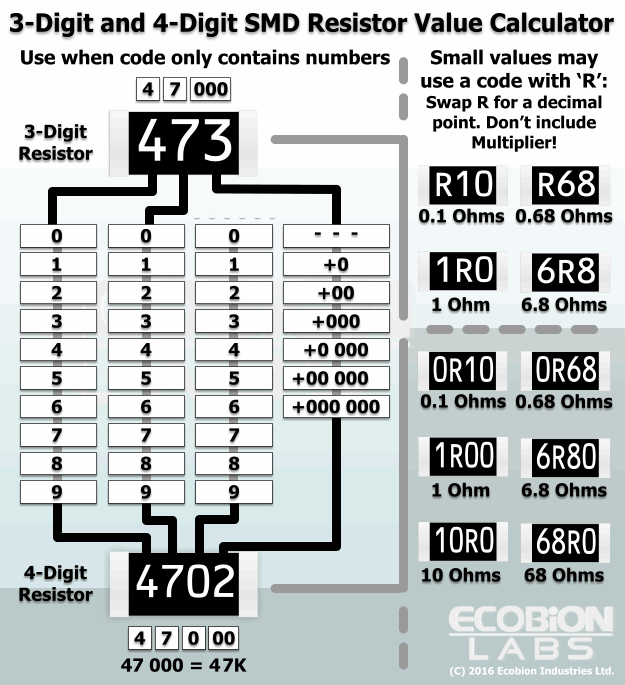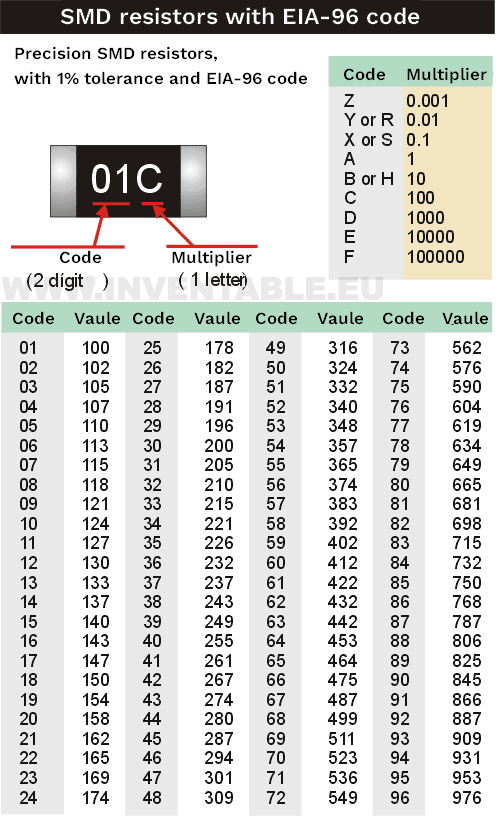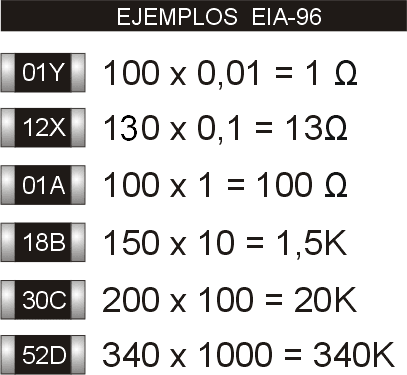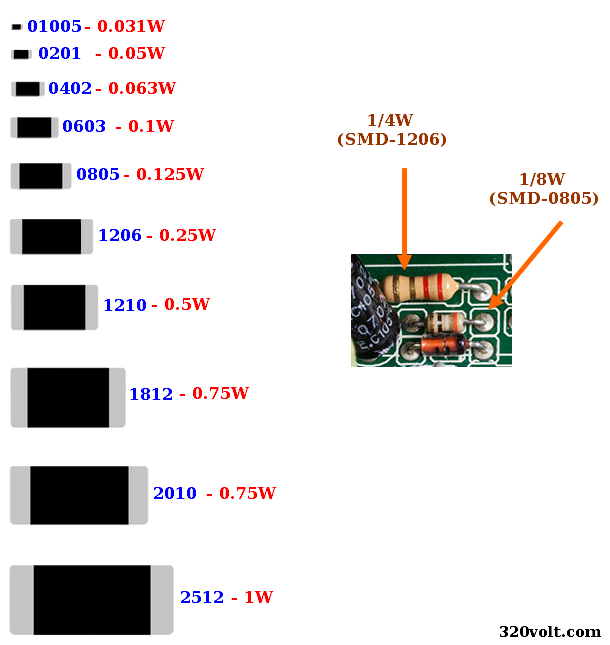# Calculating SMD Resistor Value

 Enter SMD Resistor Vaule:

3 digit
4 digit
EIA-96

In addition to the SMD resistor calculation application above, information about SMD resistor codes… SMD resistor codes are the same as the calculation of DIP resistors. The numerical values of the colors are written directly on the SMD resistors.

### 3-digit SMD Resistor codes

As with the 3-color DIP sheath resistor codes, the first 2 digits are the numerical value, and the third digit is the multiplier, that is, the number of zeros we need to add to the value. If the multiplier is “0”, the first numerical values ​​are valid. Their tolerance is 5%.

Example: 332 is written on the SMD resistor, we convert the last digit, that is, two, to zero, it becomes 3300, that is, 3300 OHM bin OHM is 1k, so 3300-OHM is 3.3K. 330 is written on the resistor, if the first 2 digits are numerical, the third is not taken into account because the multiplier is “0”, it is ineffective. In this case, the resistor value is 33-OHM.

For fractional OHM values ​​in SMD resistors with a 3-digit code, comma and “R” are added instead of dots.

Example: 4.7-OHM is written on the resistor as 4R7.

In low value resistors such as 0.22-OHM, 0.47-OHM, “R” is added to the beginning R22 : 0.22-OHM R47 : 0.47-OHM

### 4-digit SMD Resistor codes

In 4-digit SMD resistors, the first three digits are the numerical value, the fourth digit is the multiplier, that is, the number of zeros we need to add to the value. If the multiplier is “0”, the first numerical values ​​are valid. Their tolerance is 1%.

Example: 1001 is written on the SMD resistor, the last digit is one, we convert it to zero, it becomes 100 + 0, that is, 1000-OHM bin OHM 1k, that is, the value of our resistor is 1K on the resistor. value 100-OHM

In SMD resistors with a 4-digit code, “R” is used for fractional OHM values ​​and values ​​below 100-OHM.

Example: 4R70 for 4.7OHM writes 47R0 for 47-OHM.

Note: SMD resistors with only “0” zero on them have no value, direct short circuit, that is, jumper is used instead of jumper wire. It can withstand instantaneous high currents according to their power. According to the datasheet information of Yeago company, the rated current over the 0805 sheath SMD resistor; 2A max.; It is said that it can pass 5A current

3 and 4 digit SMD resistor codes calculation tableecobionlabs.com

### EIA-96 coded SMD resistors

Manufacturers have developed a new code system called EIA-96. The first two digits of the number read in EIA-96 are values, the last letter is a multiplier.inventable.eu/

### SMD Resistor Power

In the past, the most used type of SMD resistor was 1206 sheath, that is, the standard 1/4W type found in all electronic equipment, after which 0805 and much smaller cases began to be used. The table shows the power of SMD resistors and the most used 1/4w, 1/8w resistance comparison.## 1 Comment “Calculating SMD Resistor Value”

1.Mirceacluj

Very practic